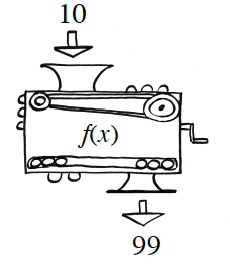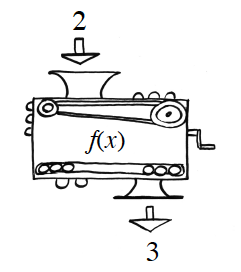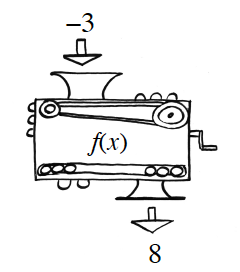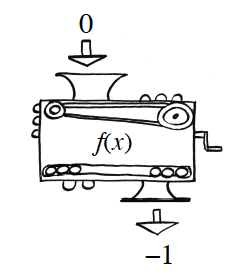### Home > INT2 > Chapter 9 > Lesson 9.1.3 > Problem9-29

9-29.

Each of the following pictures shows how the same function machine changes the given $x$-value into a corresponding $f(x)$-value. What is the equation for this machine?Copy and complete the table for the function.

 $x$ $10$ $2$ $-3$ $f(x)$ $99$ $3$

$f(x) = x^2 - 1$